# Benchmarking the speed of fold and SKTime.## Installing libraries, defining utility functions

``````%%capture
pip install --quiet fold-core fold-models krisi fold-wrappers matplotlib seaborn xgboost plotly prophet statsforecast statsmodels ray kaleido sktime pmdarima
``````
``````from time import monotonic
import pandas as pd
from collections import defaultdict
from krisi import score
from krisi.report import plot_y_predictions
import plotly.io as pio
pio.renderers.default = "png"

class Timing:
results = defaultdict(lambda: defaultdict(dict))

def record_time(self, model_name: str, framework: str):
def wrapper( function, *args, **kwargs):
start_time = monotonic()
return_value = function(*args, **kwargs)
print(f"Run time: {monotonic() - start_time} seconds")

self.results[framework][model_name] = monotonic() - start_time
return return_value
return wrapper
def summary(self):
pd.set_option('display.max_rows', None)
pd.set_option('display.max_columns', None)
pd.set_option('display.precision', 3)
display(pd.DataFrame(self.results))

timing = Timing()

def flatten_result_windows(series: pd.Series) -> pd.Series:
return pd.concat(series.to_list())
``````

``````from fold.utils.dataset import get_preprocessed_dataset

X, y = get_preprocessed_dataset(
"weather/historical_hourly_la", target_col="temperature", resample="H", shorten=1000
)

pd.concat([y,X], axis='columns').plot(subplots=True);
``````
``````                     humidity  pressure  wind_speed  wind_direction  \
datetime
2012-10-01 13:00:00      88.0    1013.0         0.0             0.0
2012-10-01 14:00:00      88.0    1013.0         0.0             0.0
2012-10-01 15:00:00      88.0    1013.0         0.0             0.0
2012-10-01 16:00:00      88.0    1013.0         0.0             0.0
2012-10-01 17:00:00      88.0    1013.0         0.0             0.0

temperature
datetime
2012-10-01 13:00:00   291.870000
2012-10-01 14:00:00   291.868186
2012-10-01 15:00:00   291.862844
2012-10-01 16:00:00   291.857503
2012-10-01 17:00:00   291.852162
datetime
2012-10-01 13:00:00    291.868186
2012-10-01 14:00:00    291.862844
2012-10-01 15:00:00    291.857503
2012-10-01 16:00:00    291.852162
2012-10-01 17:00:00    291.846821
Freq: H, Name: temperature, dtype: float64
``````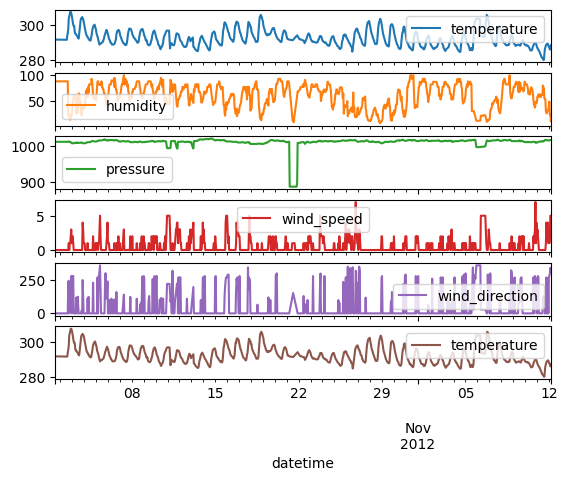``````# Default values that both sktime and fold will receive

step_size = 50
initial_train_size = 300
lag_length_for_tabular_models = 10
fh=list(range(1, step_size+1))
``````

## SKTime - Long forecasting horizon (Time Series Cross-Validation)

``````from sktime.forecasting.model_evaluation import evaluate
from sktime.forecasting.model_selection import ExpandingWindowSplitter as SKTimeExpandingWindowSplitter
from sktime.forecasting.naive import NaiveForecaster
from sktime.forecasting.arima import ARIMA
from sktime.pipeline import make_pipeline
from sktime.forecasting.compose import make_reduction
``````
``````cv = SKTimeExpandingWindowSplitter(initial_window=initial_train_size, step_length=step_size, fh=fh)
``````

### Naive

``````forecaster = NaiveForecaster(strategy="last")
results = timing.record_time('naive', 'sktime (long-fh)')(evaluate, forecaster=forecaster, y=y, X=X, cv=cv, return_data=True, error_score='raise')
predictions = flatten_result_windows(results['y_pred']).rename("naive")
plot_y_predictions(y[predictions.index], predictions.to_frame(), mode="overlap")
score(y[predictions.index], predictions)[['rmse']].print('minimal')
``````
``````Run time: 0.32869669699999804 seconds
``````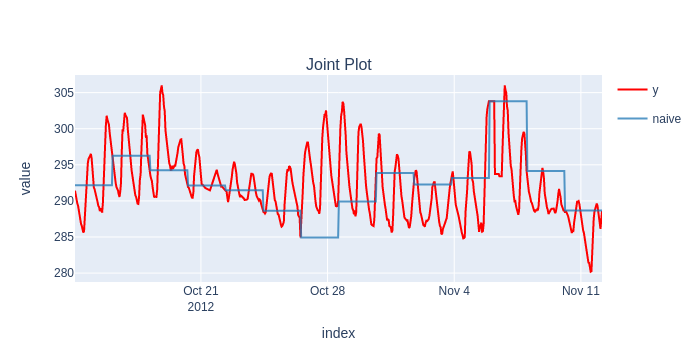```                                            naive
Root Mean Squared Error  5.36894
```

### Statsmodels ARIMA

``````forecaster = ARIMA((1,1,0))

results = timing.record_time('arima', 'sktime (long-fh)')(evaluate, forecaster=forecaster, y=y, cv=cv, return_data=True, error_score='raise')
predictions = flatten_result_windows(results['y_pred'])
plot_y_predictions(y[predictions.index], predictions.to_frame(), mode="overlap")
score(y[predictions.index], predictions)[['rmse']].print('minimal')
``````
``````Run time: 2.449221571999999 seconds
``````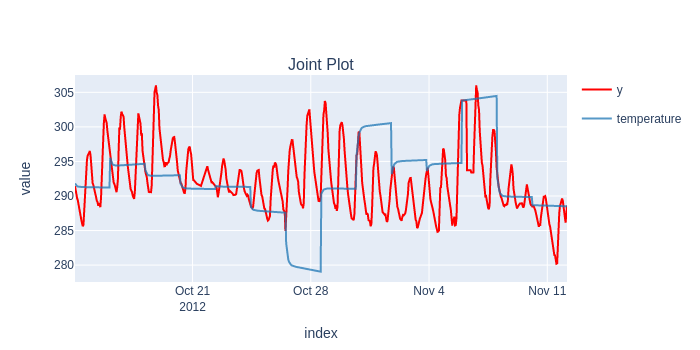```                                          temperature
Root Mean Squared Error     6.743475
```

A seasonal ARIMA - not suprisingly - provides much better results, but because of the slowness (and out of memory errors), we couldn't benchmark Statsmodels' implementation.

### XGBoost

``````from xgboost import XGBRegressor
regressor = XGBRegressor(random_state=42)

forecaster = make_reduction(regressor, strategy="recursive", window_length=lag_length_for_tabular_models)
results = timing.record_time('xgboost', 'sktime (long-fh)')(evaluate, forecaster=forecaster, y=y, X=X, cv=cv, backend="multiprocessing", return_data=True, error_score='raise')
predictions = flatten_result_windows(results['y_pred'])
plot_y_predictions(y[predictions.index], predictions.to_frame(), mode="overlap")
score(y[predictions.index], predictions)[['rmse']].print('minimal')
``````
``````Run time: 44.59098899100002 seconds
``````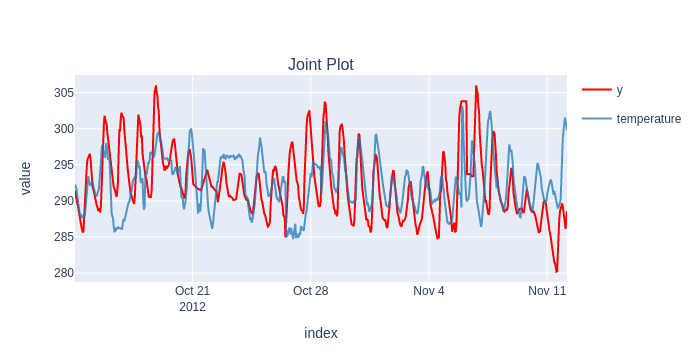```                                          temperature
Root Mean Squared Error     5.044424
```

### Results

``````timing.summary()
``````
sktime (long-fh)
arima 2.449
naive 0.329
xgboost 44.591

SKTime may look fast on its own, but it definitely falls short when it comes to the real usefulness of Time Series Cross-Validation.

The models are static, stuck in the past between end of the training windows, they don't have access to the latest value, and therefore their predictions are way off.

## Fold - Short forecasting horizon (Continuous Validation)

fold has the ability to update models within the test window, in an "online" manner:``````from fold import train_evaluate, ExpandingWindowSplitter, BackendType
from fold.models.wrappers import WrapXGB, WrapStatsModels
from fold.models import Naive
from statsmodels.tsa.arima.model import ARIMA as StatsModelARIMA
import ray
ray.init(ignore_reinit_error=True)
``````
``````2023-04-17 11:07:04,950 INFO worker.py:1553 -- Started a local Ray instance.
``````

### Ray

 Python version: 3.9.16 Ray version: 2.3.1
``````splitter = ExpandingWindowSplitter(initial_train_window=initial_train_size, step=step_size)
``````

### Naive

``````model = Naive()

scorecard, predictions, _ = timing.record_time('naive', 'fold (online)')(train_evaluate, model, None, y, splitter, backend=BackendType.no, silent=True)
plot_y_predictions(y[predictions.index], predictions, mode="overlap")
scorecard[['rmse']].print('minimal')
``````
``````Run time: 0.17832120899998927 seconds
``````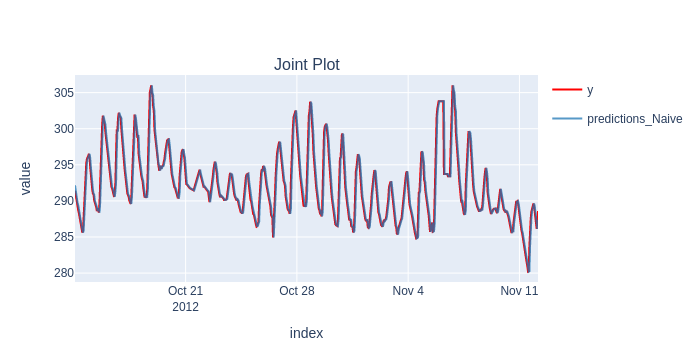```                                          predictions_Naive
Root Mean Squared Error        1.224
```

### Statsmodels ARIMA (Online)

``````model = WrapStatsModels(StatsModelARIMA, init_args=dict(order=(1, 1, 0)), online_mode=True)
scorecard, predictions, _ = timing.record_time('arima', 'fold (online)')(train_evaluate, model, None, y, splitter, backend=BackendType.no, silent=True)
plot_y_predictions(y[predictions.index], predictions, mode="overlap")
scorecard[['rmse']].print('minimal')
``````
``````Run time: 41.32513004100002 seconds
``````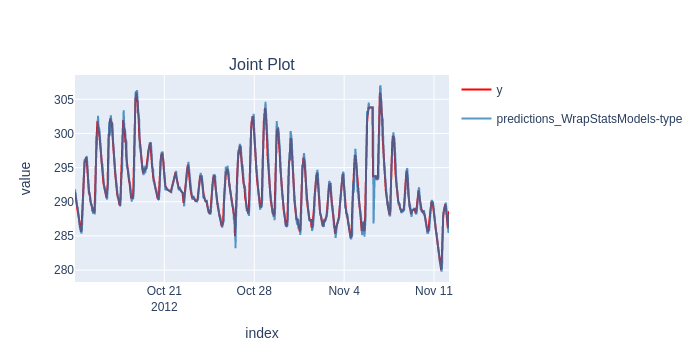```                                          predictions_WrapStatsModels-type
Root Mean Squared Error                0.927
```

### XGBoost

``````from xgboost import XGBRegressor
model = XGBRegressor(random_state=42)
pipeline = [AddLagsX(("all", list(range(lag_length_for_tabular_models))) ), model]

scorecard, predictions, _ = timing.record_time('xgboost', 'fold (online)')(train_evaluate, pipeline, X, y, splitter, backend=BackendType.ray, silent=True)
plot_y_predictions(y[predictions.index], predictions, mode="overlap")
scorecard[['rmse']].print('minimal')
``````

This results in much more realistic simulation of past performance (in case the last value is available in production).

### Results

``````timing.summary()
``````
sktime (long-fh) fold (online)
naive 0.329 0.180
arima 2.449 41.325
xgboost 44.591 13.256

And it's also substantially faster, except in the case of Statsmodels' ARIMA, which `fold` needs to update on every timestamp. Our own ARIMA (coming in April) will provide a ca. 100x speedup here.

## SKTime - Short forecasting horizon (Continuous Validation)

``````cv = SKTimeExpandingWindowSplitter(initial_window=initial_train_size, step_length=1, fh=1)
``````

Now let's see what SKTime's training speed would be like if we wanted to replicate "Continuous Validation", and the models having access to the latest value within the folds.

This means we'll need to update (not possible with the tested models) or fit a new model for every timestamp we return.

### Naive

``````forecaster = NaiveForecaster(strategy="last")
results = timing.record_time('naive', 'sktime (online)')(evaluate, forecaster=forecaster, y=y, X=X, cv=cv, return_data=True, strategy="refit", error_score='raise')
predictions = flatten_result_windows(results['y_pred']).rename("naive")
plot_y_predictions(y[predictions.index], predictions.to_frame(), mode="overlap")
score(y[predictions.index], predictions)[['rmse']].print('minimal')
``````
``````Run time: 18.046849753999993 seconds
``````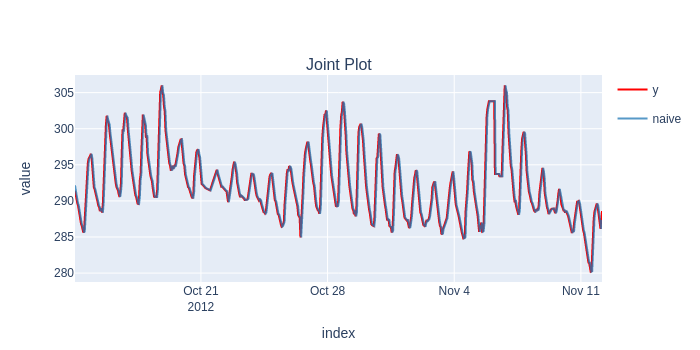```                                          naive
Root Mean Squared Error  1.224
```

### Statsmodels ARIMA

``````from sktime.forecasting.arima import ARIMA
forecaster = ARIMA((1,1,0))

results = timing.record_time('arima', 'sktime (online)')(evaluate, forecaster=forecaster, y=y, cv=cv, return_data=True, error_score='raise')
predictions = flatten_result_windows(results['y_pred'])
plot_y_predictions(y[predictions.index], predictions.to_frame(), mode="overlap")
score(y[predictions.index], predictions)[['rmse']].print('minimal')
``````
``````/usr/local/lib/python3.9/dist-packages/statsmodels/base/model.py:604: ConvergenceWarning:

Maximum Likelihood optimization failed to converge. Check mle_retvals

Run time: 106.65218957599996 seconds
``````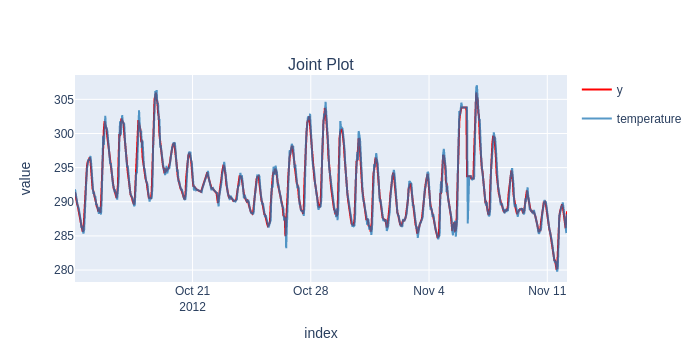```                                          temperature
Root Mean Squared Error     0.927
```

### XGBoost

``````from xgboost import XGBRegressor
regressor = XGBRegressor(random_state=42)

forecaster = make_reduction(regressor, strategy="recursive", window_length=lag_length_for_tabular_models)
results = timing.record_time('xgboost', 'sktime (online)')(evaluate, forecaster=forecaster, y=y, X=X, cv=cv, backend=None, return_data=True, error_score='raise')
predictions = flatten_result_windows(results['y_pred'])
plot_y_predictions(y[predictions.index], predictions.to_frame(), mode="overlap")
score(y[predictions.index], predictions)[['rmse']].print('minimal')
``````
``````Run time: 759.161927353 seconds
``````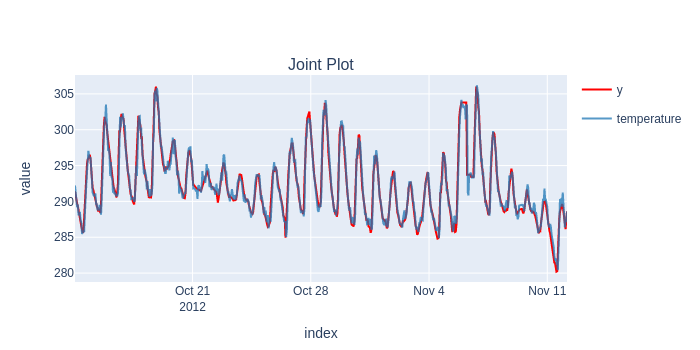```                                          temperature
Root Mean Squared Error     1.018
```

## Overall Results

``````timing.summary()
``````
sktime (long-fh) fold (online) sktime (online)
naive 0.329 0.180 18.048
arima 2.449 41.325 106.653
xgboost 44.591 13.256 759.163

Overall, `fold` provides a speedup between 3x and 100x, already.

When it comes to practice, we argue that this makes Continuos Validation feasible, compared to other tools out there.